# SN Dey Class 11 Solutions Differentiation Short Answer Type Questions

## SN Dey Class 11 Differentiation Short Answer Type Questions Solutions

SN Dey class 11 Differentiation short answer type questions Ex 1 solutions:

Ex 1: Examine whether f(x)=|x+1| has a derivative at x=-1.

Solution: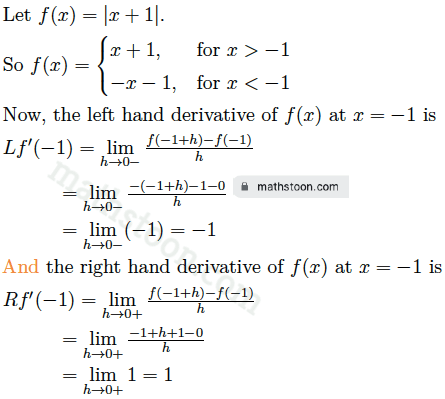As $Lf'(-1) \neq Rf'(-1)$, we conclude that f(x)=|x+1| has no derivative at x=-1.

SN Dey class 11 Differentiation short answer type questions Ex 2 solutions:

Ex 2: If the derivative of f(x) at x=a is $f'(a)$, show that

$\lim\limits_{x \to a}\dfrac{xf(a)-af(x)}{x-a}$ $=f(a)-af(a)$

Solution: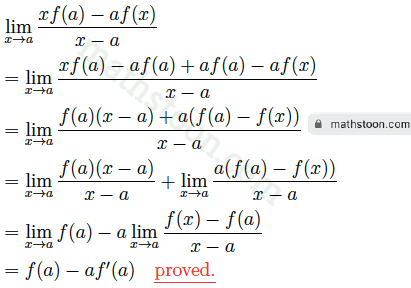SN Dey class 11 Differentiation short answer type questions Ex 3 solutions:

Ex 3: Find from the first principle, the derivatives of the following function:

Ex 3.(i): From the first principle, find the derivative of x3.

Solution: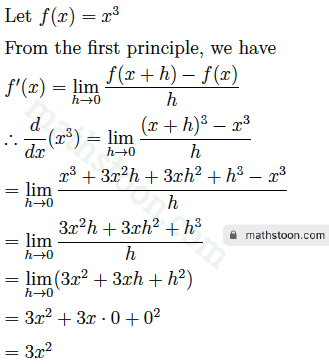Ex 3.(ii): From the first principle, find the derivative of x6.

Solution: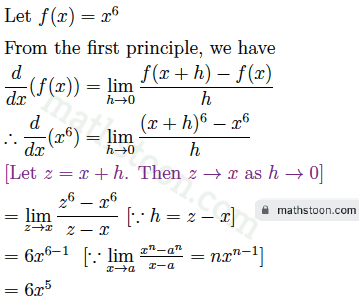Derivative of square root of x : The derivative of root x is 1/2√x.

Ex 3.(iii): From the first principle, find the derivative of √x.

Solution: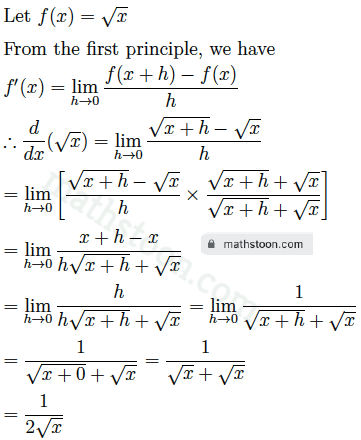Ex 3.(iv): From the first principle, find the derivative of tan $\frac{x}{2}$.

Solution: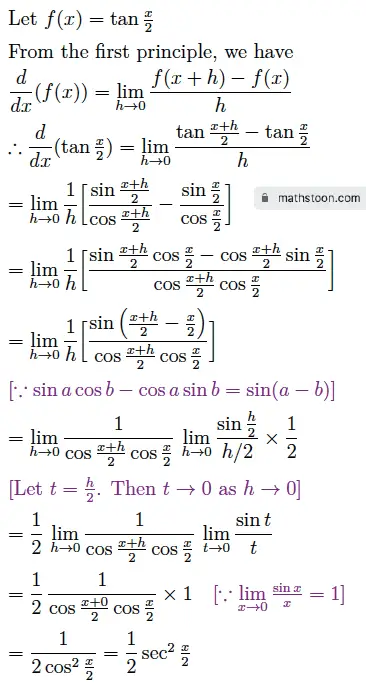Ex 3.(v): From the first principle, find the derivative of sec 3x.

Solution: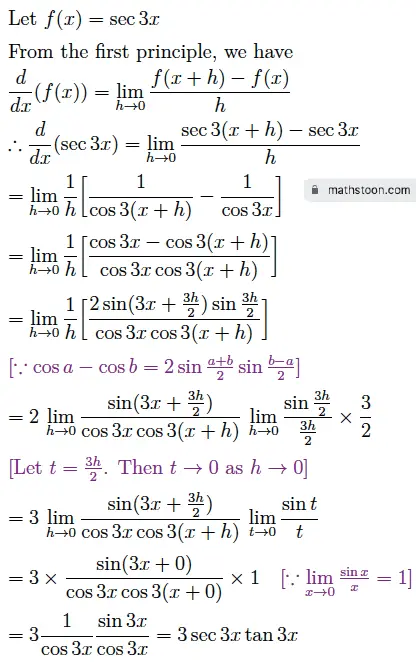Ex 3.(vi): From the first principle, find the derivative of sin 4x.

Solution: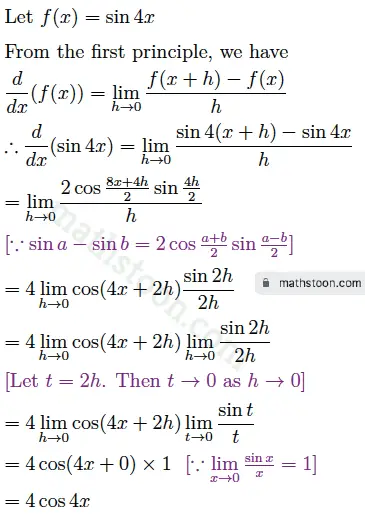Ex 3.(vii): From the first principle, find the derivative of sin x°.

Solution: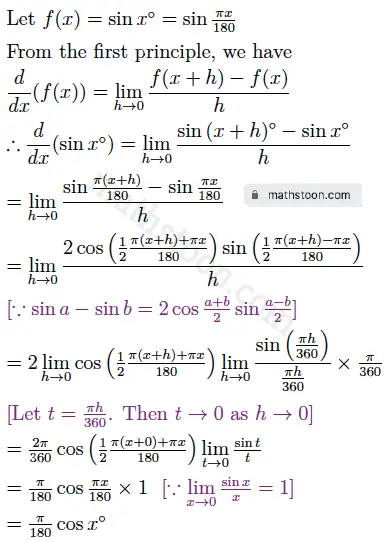Ex 3.(viii): From the first principle, find the derivative of 1/√x.

Solution: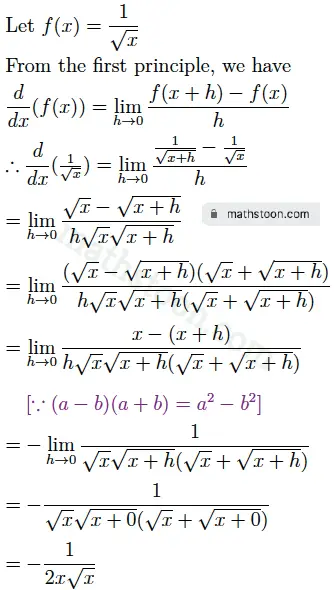Ex 3.(ix): From the first principle, find the derivative of e3x.

Solution: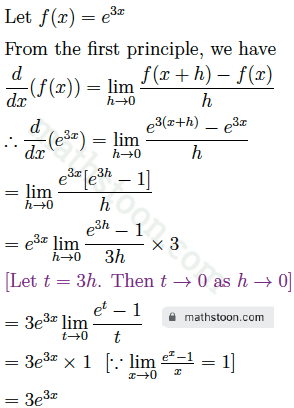Ex 3.(x): From the first principle, find the derivative of 3√x.

Solution: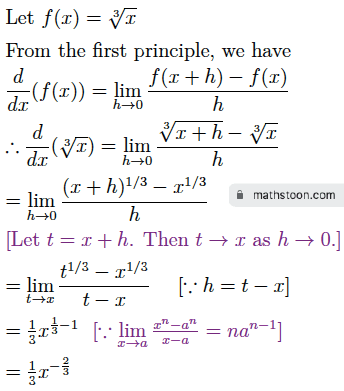Ex 3.(xi): From the first principle, find the derivative of log 3x.

Solution: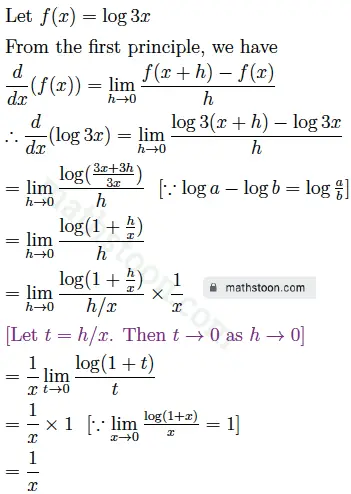Ex 4: Find from the definition of the differential coefficients of the following function:

Ex 4.(i): y=x+$\frac{1}{x}$ at x=1.

Solution: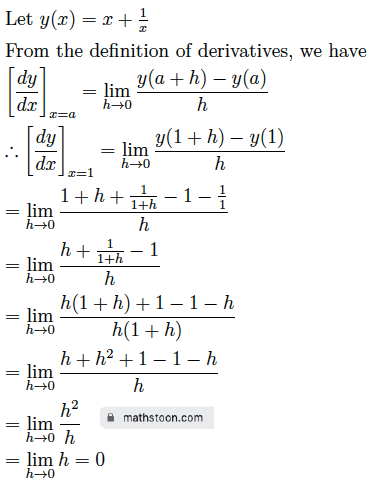Ex 4.(ii): y=4 at x=3.

Solution: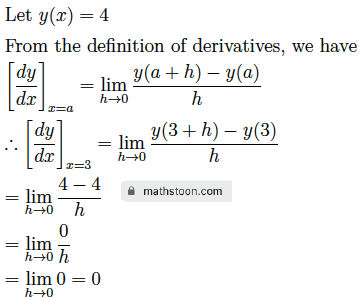Ex 4.(iii): y=$\frac{1}{2x+3}$ at x=0.

Solution: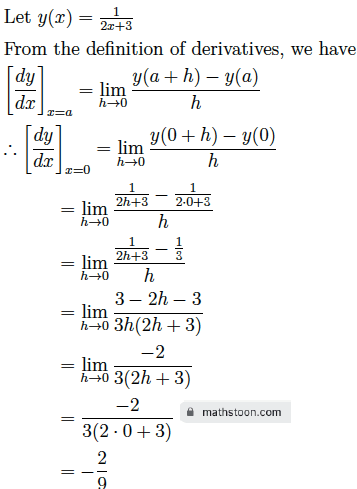Ex 4.(iv): y=sec 2x at x=$\frac{\pi}{6}$.

Solution: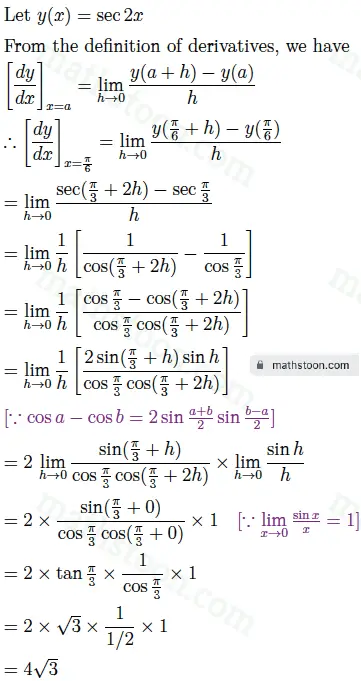Ex 4.(v): y=√x at x=2.

Solution: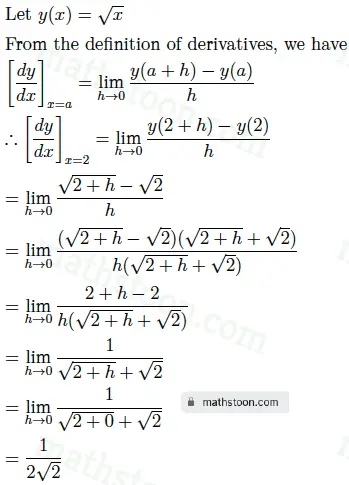Ex 4.(vi): y=x2/3 at $x=\frac{1}{27}$.

Solution: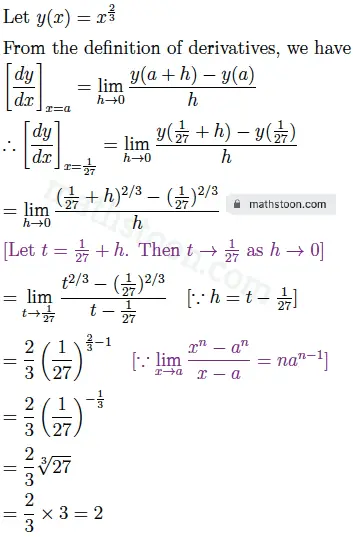Ex 4.(vii): y=e-2x at x=0.

Solution: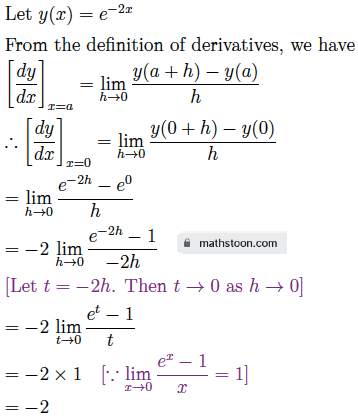Ex 4.(viii): y=cot $\frac{x}{2}$ at x=$\pi$.

Solution: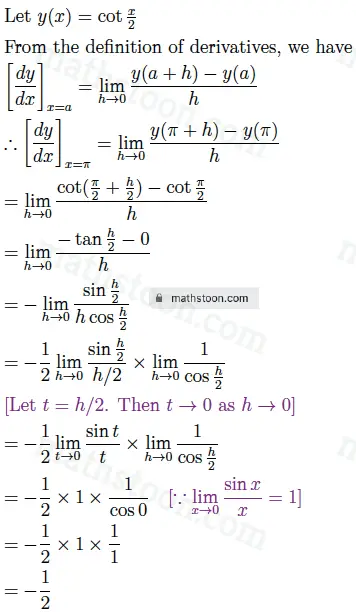Ex 4.(ix): y=cos 2x at x=$\frac{\pi}{4}$.

Solution: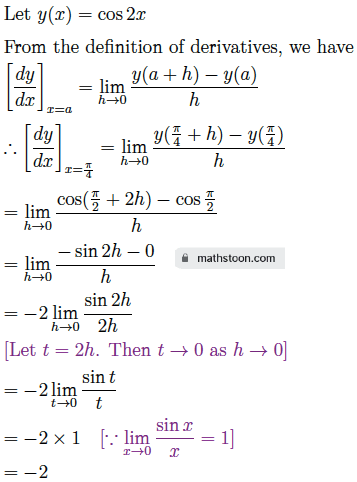Ex 6: Differentiate the following functions with respect to x:

Ex 6.(i): 10x ⋅ x10

Solution: The derivative of 10x ⋅ x10 is equal to

d/dx(10x ⋅ x10) = 10x d/dx(x10) + x10 d/dx(10x) , by the product rule of derivatives.

= 10x ⋅ 10x10-1 + x10⋅10x loge10

= 10x+1 ⋅x9 + x10⋅10x loge10

Ex 6.(ii): x3log x

Solution:

d/dx(x3 log x) = x3 d/dx(log x) + log x d/dx(x3

= x3 ⋅ 1/x + log x ⋅ 3x2

= x2 + 3x2 log x

= x2 (1 + 3log x)

Ex 6.(iii): etan x

Solution: The derivative of etan x is equal to

d/dx(etan x) = ex d/dx(tan x) + tan x d/dx(ex) , by the product rule of derivatives.

= ex ⋅ sec2x + tan x ⋅ ex

= e(tan x + sec2x)

Ex 6.(iv): √x log √x

Solution:

d/dx(√x log √x) = √x ⋅ d/dx(log √x) + log √x ⋅ d/dx(√x)

$=\sqrt{x} \frac{1}{\sqrt{x}} \frac{d}{dx}(\sqrt{x})$ $+\log \sqrt{x} \frac{d}{dx}(\sqrt{x})$

$=\frac{d}{dx}(x^{1/2}) (1+\log \sqrt{x})$

$=\frac{1}{2} x^{1/2-1} (1+\log \sqrt{x})$

$=\frac{1}{2\sqrt{x}} (1+\log \sqrt{x})$

Ex 6.(v): (x2+1)ex

Solution:

d/dx{(x2+1)ex} = (x2+1) d/dx(ex) + ex d/dx(x2+1)

= (x2+1) ex + ex (2x+0)

= ex (x2+1+2x)

= ex (x+1)2

Ex 6.(vi): ex sec x

Solution:

The derivative of ex sec x is equal to

d/dx(ex sec x) = ex d/dx(sec x) + sec x d/dx(ex) , by the product rule of derivatives.

= ex ⋅ sec x tan x + sec x ⋅ ex

= esec x (tan x + 1)

Ex 6.(vii): (2x-5)(x2+2)

Solution:

Ex 6.(viii): cosec x ⋅ cot x

Solution:

The derivative of cosec x ⋅ cot x is equal to

d/dx(cosec x ⋅ cot x) = cosec x d/dx(cot x) + cot x d/dx(cosec x) , by the product rule of derivatives.

= cosec x ⋅ (-cosec2 x) + cot x ⋅ (-cosec x cot x)

= -cosec x (cosec2x+cot2x)

Ex 6.(ix): (sin x +sec x)(cos x +cosec x)

Solution:

The derivative of (sin x +sec x)(cos x +cosec x) is equal to

d/dx[(sin x +sec x)(cos x +cosec x)]

= (sin x +sec x) d/dx(cos x +cosec x) + (cos x +cosec x) d/dx(sin x +sec x)

= (sin x +sec x) (-sin x -cosec x cot x) + (cos x +cosec x) (cos x +sec x tan x)

Ex 6.(x): sec3 x

Solution: The derivative of sec3x is equal to

d/dx(sec3 x) = 3 sec2 x ⋅ d/dx(sec x)

= 3 sec2 x ⋅ sec x tan x

= 3 sec3 x tan x

Ex 6.(xi): √xesec x

Solution:

Ex 6.(xii): xtan x log x

Solution:

Ex 6.(xiii): x cos x + 2x sec x

Solution:

The derivative of x cos x + 2x sec x is equal to

d/dx(x cos x + 2x sec x) = d/dx(x cos x) + d/dx(2x sec x)

= x d/dx(cos x) + cos x d/dx(x) + 2x d/dx(sec x) + sec x d/dx(2x)

= x sin x + cos x ⋅ 1 + 2x sec x tan x + sec x ⋅ 2xloge2

= x sin x + cos x + 2x sec x (tan x + loge2)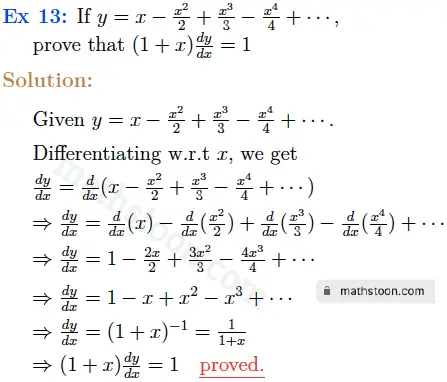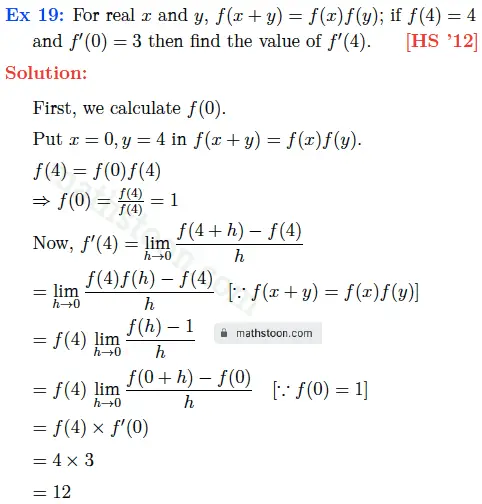Keywords: SN Dey Class 11 Differentiation Solutions | Solution of Class 11 Derivative | SN Dey Class 11 Solutions | SN Dey Class 11 Derivative Solutions Short Answer Type Questions | WB Board Class 11 Derivative Solution | SN Dey Class 11 Math Chapter Derivative Solution | Differentiation Short Answer Type Questions Answers | sn dey class 11 derivatives solutions

Share via: